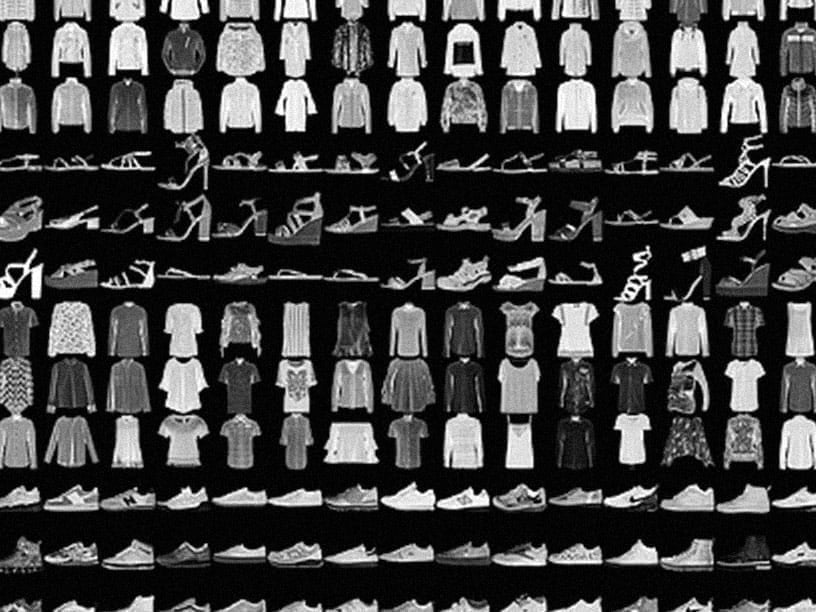Stay organized with collections Save and categorize content based on your preferences.

# TensorFlow is an end-to-end open source platform for machine learning

TensorFlow makes it easy for beginners and experts to create machine learning models. See the sections below to get started.#### For beginners

The best place to start is with the user-friendly Sequential API. You can create models by plugging together building blocks. Run the “Hello World” example below, then visit the tutorials to learn more.

To learn ML, check out our education page . Begin with curated curriculums to improve your skills in foundational ML areas.

```import tensorflow as tf
mnist = tf.keras.datasets.mnist

(x_train, y_train),(x_test, y_test) = mnist.load_data()
x_train, x_test = x_train / 255.0, x_test / 255.0

model = tf.keras.models.Sequential([
tf.keras.layers.Flatten(input_shape=(28, 28)),
tf.keras.layers.Dense(128, activation='relu'),
tf.keras.layers.Dropout(0.2),
tf.keras.layers.Dense(10, activation='softmax')
])

loss='sparse_categorical_crossentropy',
metrics=['accuracy'])

model.fit(x_train, y_train, epochs=5)
model.evaluate(x_test, y_test)
```

#### For experts

The Subclassing API provides a define-by-run interface for advanced research. Create a class for your model, then write the forward pass imperatively. Easily author custom layers, activations, and training loops. Run the “Hello World” example below, then visit the tutorials to learn more.

```class MyModel(tf.keras.Model):
def __init__(self):
super(MyModel, self).__init__()
self.conv1 = Conv2D(32, 3, activation='relu')
self.flatten = Flatten()
self.d1 = Dense(128, activation='relu')
self.d2 = Dense(10, activation='softmax')

def call(self, x):
x = self.conv1(x)
x = self.flatten(x)
x = self.d1(x)
return self.d2(x)
model = MyModel()

with tf.GradientTape() as tape:
logits = model(images)
loss_value = loss(logits, labels)
```

## Solutions to common problemsFor beginners
Your first neural network

Train a neural network to classify images of clothing, like sneakers and shirts, in this fast-paced overview of a complete TensorFlow program.For experts

Train a generative adversarial network to generate images of handwritten digits, using the Keras Subclassing API.For experts
Neural machine translation with attention

Train a sequence-to-sequence model for Spanish to English translation using the Keras Subclassing API.

## News & announcements

Check out our blog for additional updates, and subscribe to our TensorFlow newsletter to get the latest announcements sent directly to your inbox.

## Community participation

See more ways to participate in the TensorFlow community.

[{ "type": "thumb-down", "id": "missingTheInformationINeed", "label":"Missing the information I need" },{ "type": "thumb-down", "id": "tooComplicatedTooManySteps", "label":"Too complicated / too many steps" },{ "type": "thumb-down", "id": "outOfDate", "label":"Out of date" },{ "type": "thumb-down", "id": "samplesCodeIssue", "label":"Samples / code issue" },{ "type": "thumb-down", "id": "otherDown", "label":"Other" }]
[{ "type": "thumb-up", "id": "easyToUnderstand", "label":"Easy to understand" },{ "type": "thumb-up", "id": "solvedMyProblem", "label":"Solved my problem" },{ "type": "thumb-up", "id": "otherUp", "label":"Other" }]